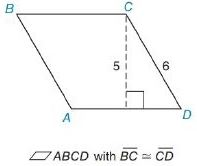Chapter 8.2, Problem 16E### Elementary Geometry for College St...

6th Edition
Daniel C. Alexander + 1 other
ISBN: 9781285195698

#### Solutions

Chapter
Section### Elementary Geometry for College St...

6th Edition
Daniel C. Alexander + 1 other
ISBN: 9781285195698
Textbook Problem
1 views

# In Exercises 13 to 18, find the area of the given polygon.To determine

To Find:

Area of the parallelogram.

Explanation

Formula Used:

The area A of a parallelogram with a base of length b and with corresponding altitude of length h is given by A=bh.

Calculation:

It is given that BC¯CD¯, b=6 and h=5.

In rhombus, opposite sides are parallel and all sides are in equal length

### Still sussing out bartleby?

Check out a sample textbook solution.

See a sample solution

#### The Solution to Your Study Problems

Bartleby provides explanations to thousands of textbook problems written by our experts, many with advanced degrees!

Get Started

#### Prove the identity. 50. 2 csc 2t = sec t csc t

Single Variable Calculus: Early Transcendentals

#### Simplify the expressions in Exercises 97106. (xy)1/3(yx)2/3

Finite Mathematics and Applied Calculus (MindTap Course List)

#### Are the following graphs of functions? Explain. a. b. c. d.

Applied Calculus for the Managerial, Life, and Social Sciences: A Brief Approach